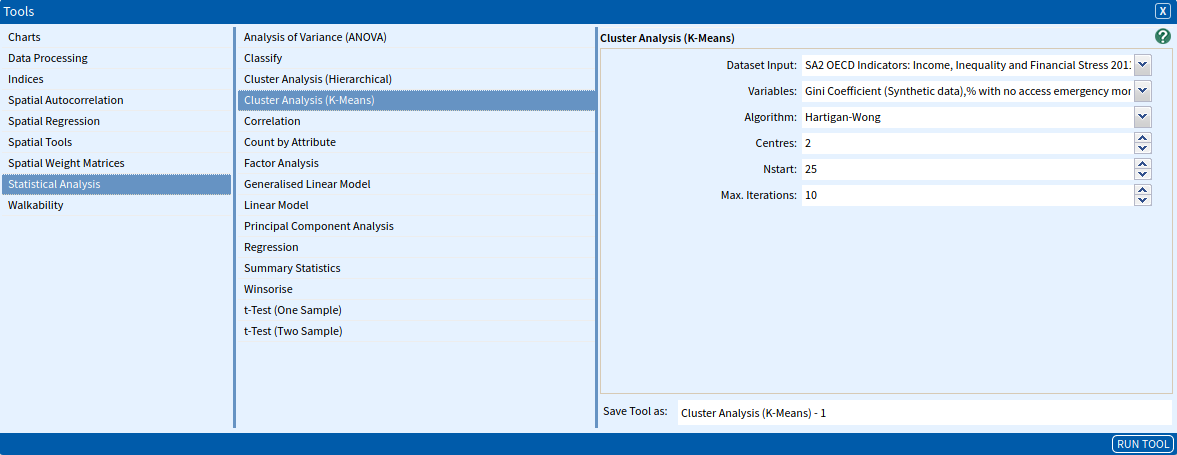# Cluster Analysis (K-Means)

Cluster Analysis (K-Means) aims to partition the numeric matrix of data points into $k$-groups such that the sum of squares from data points to the assigned cluster centres is minimised. At the minimum, all cluster centres are at the mean of their Voronoi sets (the set of data points which are nearest to the cluster centre).

The following algorithm is used in the implementation of the K-Means Clustering.

• Randomly select K cluster centres
• Repeat the following until there is convergence or the maximum number of iterations has occurred:
• Assigning data points to the nearest cluster centre with the sum of squares from data points to the cluster centres is minimised.
• Re-randomly select K cluster centres.

### SET UP

To illustrate the use of the Clustering Analysis (K-Mean) tool, we will use a dataset with a number of variables in it that can be related to each other: Income, Inequality and Financial Stress across the Greater Hobart area. To do this:

• Select Greater Hobart as your area
• Select SA2 OECD Indicators: Income, Inequality and Financial Stress 2011 as your dataset, selecting all variables.

### Inputs

Once you have done this, open the Hierarchical Clustering (K-Mean) tool (Tools → Statistical Analysis→ Cluster Analysis (K-Means)) and enter the parameters as listed below:

• Dataset Input: The dataset that contains the variables of interest. Select SA2 OECD Indicators: Income, Inequality and Financial Stress 2011.
• Variables: A set of independent variables to be used as the predictor(s) in the analysis. Select the following five attributes:
• Median Disposable Income (Synthetic Data)
• Gini Coefficient (Synthetic Data)
• Poverty Rate (Synthetic Data)
• % Can’t afford a night out (Synthetic Data)
• Algorithm: This is the algorithm to be used according to the respective implementations in the literature. Refer to the reference list at the bottom of the guide for further information. Select Hartigan-Wong. The options available to select from are:
• Hartigan-Wong, from Hartigan & Wong (1979).
• Lloyd, from Lloyd (1982).
• Forgy, from Forgy (1965).
• MacQueen, from MacQueen (1967).
• Centres: The number of distinct cluster-centres chosen randomly in the dataset. Select 2.
• Nstart: The number of random sets to be chosen. Select 25.
• Max. Iterations: The maximum number of iterations allowed. Select 10.Once you have selected your parameters, click the Run Tool button.

### Outputs

Once you have run the tool, click the Display Output button which appears in the pop-up dialogue box. This should open up a textual output looking like the one shown below. The text is tab-delimited and can be imported by copying and pasting, or by clicking the Download button and importing into a spreadsheet program.The above output includes the following:

• Clustering vector indices, classes: A vector of integers from $1:k$, indicating the cluster to which each data point is allocated.
• Cluster means: A matrix of cluster centres (means).
• Total cluster ss (sum of squares): The total sum of squares.
• Within cluster ss (sum of squares) by cluster: Vector of within-cluster sum of squares, one component per cluster.
• Total within cluster ss (sum of squares): Total within-cluster sum of squares.
• Between cluster ss (sum of squares): The between-cluster sum of squares.
• K-Means clustering with # of sizes: The number of data points in each of # clusters.
Aldenderfer, M. S., & Blashfield, R. K. (1984). Cluster analysis. In Quantitative applications in the social sciences (Vol. 2). Sage Publications Beverly Hills.
Forgy, E. W. (1965). Cluster analysis of multivariate data: Efficiency versus interpretability of classifications. Biometrics, 21, 768–769.
Hartigan, J. A., & Wong, M. A. (1979). Algorithm AS 136: A k-means clustering algorithm. Journal of the Royal Statistical Society. Series c (Applied Statistics), 28(1), 100–108.
Lloyd, S. (1982). Least squares quantization in PCM. IEEE Transactions on Information Theory, 28(2), 129–137.
MacQueen, J. (1967). Some methods for classification and analysis of multivariate observations. Proceedings of the Fifth Berkeley Symposium on Mathematical Statistics and Probability, 1(14), 281–297.
Venables, W. N., Smith, D. M., & Team, R. C. (2014). An introduction to R: Notes on R: A programming environment for data analysis and graphics Version 3.1.0. R Core Team.

### Looking for Spatial Data?

You can browse the AURIN Data Discovery:### How can you Create Impact?